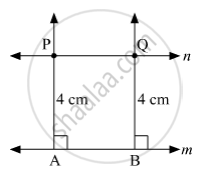SSC (English Medium) Class 8Maharashtra State Board
Share

# Draw a Line M. Draw a Line N Which is Parallel to Line M at a Distance of 4 Cm from It. - SSC (English Medium) Class 8 - Mathematics

ConceptTo Draw a Line Parallel to the Given Line

#### Question

Draw a line m. Draw a line n which is parallel to line m at a distance of 4 cm from it.

#### SolutionSteps of construction :
(1) Draw a line m.
(2) Take two points A and B on the line m.
(3) Draw perpendiculars to the line m at A and B.
(4) On the perpendicular lines, take points P and Q at a distance of 4 cm from A and B respectively.
(5) Draw a line n passing through the points P and Q.
So, line n is the required line parallel to the line m at a distance of 4 cm away from it.

Is there an error in this question or solution?

#### APPEARS IN

Balbharati Solution for Balbharati Class 8 Mathematics (2019 to Current)
Chapter 2: Parallel lines and transversals
Practice Set 2.3 | Q: 3 | Page no. 13
Solution Draw a Line M. Draw a Line N Which is Parallel to Line M at a Distance of 4 Cm from It. Concept: To Draw a Line Parallel to the Given Line.
S